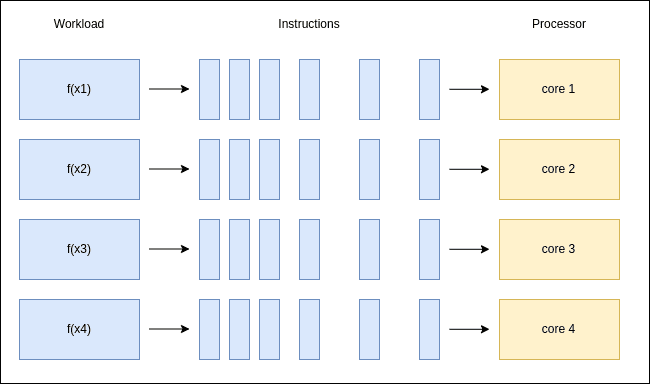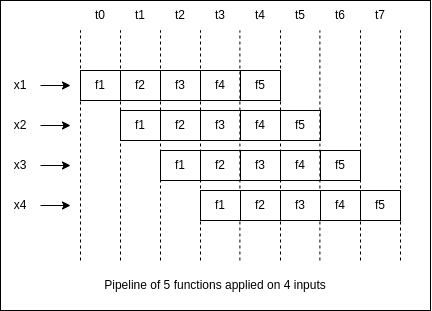## 1. Introduction

In this tutorial, we’ll study a particular property of functions called referential transparency. First, we’ll talk about how it applies to multiple programming languages and paradigms. So, we’ll also see that it has relevant ramifications on the extent to which the code can be automatically optimized.

## 2. Referential Transparency

Referential transparency is a property of a function that allows it to be replaced by its equivalent output. In simpler terms, if you call the function a second time with the same arguments, you’re guaranteed to get the same returning value.

### 2.1. Example

Let’s consider the following function, which computes the sum of 2 numbers:The function is referentially opaque because the parameters defined in its signature aren’t all of the resources the function uses.

The a and b variables are read from standard input inside the function body, so two function calls with the same arguments may produce different results.

In order to make the function referentially transparent, we need to extract the input part from the function:Note that this doesn’t prevent us from interacting with user input, we’re still able to read the values for a and b. Thus, in the code snippet, the add function is transparent, while the main function isn’t.

## 3. Relation to Programming Paradigms

As we’ve seen, if a function interacts with outside sources of information (stdin, sockets, files), it becomes referentially opaque.

Depending on the programming language we use, there are different mechanisms through which a function can interact with data other than its declared parameters.

In this section, we’ll present examples of how referential transparency can be impacted by different programming paradigms, such as imperative, object-oriented, and functional programming.

### 3.1. Imperative Programming

Imperative programming is a programming paradigm that understands programs as a sequence of instructions. It’s the manner most people initially think about coding: we define all the steps the computer has to go through to get to the desired result.

Examples: C, C++

In imperative programming, global variables and static variables can be used to store and access data across different functions. As these variables can be modified at any point, they break the referential transparency of any function that uses them.

Here’s a first example:The add function doesn’t have any parameters. However, it uses the global variables a and b. As these variables can be changed by any function, add is referentially opaque.

Here’s a second example in the C++ language:In C++, a static variable remembers its values between function calls.

In the code snippet, we’re accumulating the sum of a, b, and c into the s variable through successive calls of the add function. Because s keeps its value across function calls, add becomes referentially opaque.

### 3.2. Object-Oriented Programming

Object-Oriented programming is a programming paradigm that involves understanding our program as a collection of objects that interact with each other.

Most programming languages nowadays have object-oriented components such as classes and interfaces, as it makes it really easy to encapsulate information and behavior.

Examples: Java, C#

In object-oriented programming, the use of class attributes can also make a function referentially opaque.
For example, consider the following code:In object-oriented languages, functions can be declared inside classes, in which case they’re called methods.

The add method relies on the values stored in the class attributes a and b, which can be modified by any other method of the class. Therefore, the add method is referentially opaque.

### 3.3. Functional Programming

Lastly, functional programming is a paradigm that involves understanding our program as a composition of functions in the mathematical sense.

Functional languages actually enforce referential transparency for (almost) all of their functions. This strict limitation is offset by the many advantages that transparent functions bring, which we’ll detail in the next section.

Here’s a Haskell example that’s illustrative of what a functional program typically looks like:The main function is the only one that’s referentially opaque, all other functions are referentially transparent by design.

## 4. Use Cases

Let’s take a step back and compare how a referentially transparent function differs from a generic function.

First, it will always return the same output value for a specific set of parameters, independently of the moment it is called. Second, it won’t interact with any values outside of it, so it will neither read nor write information from other variables.

### 4.1. Caching

Caching is the mechanism of storing function results in memory for faster future retrieval.

If a function is referentially transparent, then we can save its output the first time it is called, and for each subsequent call, we only need to do a memory lookup. So, the more computationally complex the function is, the more time we save.

### 4.2. Parallelisation

Modern CPUs are built with multiple cores inside, which allow us to run multiple threads simultaneously.

If a function is referentially transparent, then we can run multiple copies of it without them interacting with one another:### 4.3. Pipelining

Most programs are longer than a simple function call. They are a sequence of function applications on a given input. For this reason, we can’t parallelize all functions in any order — we still have to keep the logical dependencies between them.

What we can do, however, is to run the sequence of functions on different inputs in an overlapping way.

If the functions in our 5-step pipeline are all referentially transparent, we can run the f1 function on a second input x2 before running the f5 function on input x1. In other words, we don’t have to wait for the pipeline to finish before starting a second one:On the other hand, if some functions are referentially opaque, they can have hidden dependencies. In this situation, we aren’t guaranteed that starting a second pipeline on another input won’t affect the output of the first pipeline.

## 5. Conclusion

In conclusion, referential transparency is a property of a function that allows it to be replaced by its equivalent output. It’s a desirable property for program optimization and is achieved by avoiding the use of a global or shared state, therefore passing all required information as parameters.

In this article, we’ve seen how referential transparency appears through the lens of different programming paradigms, including the functional paradigm which actually enforces it.